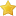Social Buttons

• 0 Vote(s) - 0 Average
• 1
• 2
• 3
• 4
• 5
 Recursive Descent in BASIC
03-20-2019, 05:29 AM
Post: #1AurelAdministratorPosts: 134 Joined: Mar 2017 Reputation: 0
Recursive Descent in BASIC
I was thinking that recursive descent in Basic as i use
in ruben interpreter work same standalone
but i found that is not Ed explained for one turn more:

so in oxygen basic i need two while loops like this:

Code:
```'recursive descent token evaluator #lookahead int tc=0 : string token string tokens tokens = "2" tokens = "*" tokens = "(" tokens = "3" tokens = "+" tokens = "4" tokens = ")" '----------------------------------------------------- sub gettok() tc++ token = tokens[tc] print "TOKEN: " + token + "  ,TC: " + str(tc) if tokens[tc+1] <> "" then return end sub '---------------------------------------------------- sub expr() as float float v If token = "-" v = -(term()) else v = term() end if while token = "+" or token = "-" if token = "+": gettok() : v = v + term(): end if if token = "-": gettok() : v = v - term(): end if wend return v end sub '--------------------------------------------------- sub term() as float float v v = factor() while token = "*" or token = "/" if token = "*": gettok() : v = v * factor(): end if if token = "/": gettok() : v = v / factor(): end if wend return v end sub '------------------------------------------------------- sub factor() as float float v if asc(token)>47  and asc(token)<58 'nums v = val(token) print str(v)+ " factor" gettok() end if if asc(token)=40 and asc(token)<>41 'match (...) gettok() : v = expr() : gettok() end if return v end sub 'execute----------------------------------------------------- gettok()'start float res = expr() print "RESULT=" + str(res)```
03-21-2019, 03:24 AM
Post: #2AurelAdministratorPosts: 134 Joined: Mar 2017 Reputation: 0
RE: Recursive Descent in BASIC
In this version i remove WHILE loops
Added var look which check one token lookahead.
and add INSTR () function to check last token if is not operator.
And looks that work in o2..
In qb64 no matter what i do without dummy load recursion
simply not work .

Code:
```'recursive descent token evaluator #lookahead int tc=0 : string token,look string tokens tokens = "2" tokens = "*" tokens = "(" tokens = "10" tokens = "-" tokens = "2" tokens = ")" 'tokens = "+" '----------------------------------------------------- sub gettok() tc++ token = tokens[tc] : look = tokens[tc+1] print "TOKEN: " + token + "  ,TC: " + str(tc) if tokens[tc+1] <> "" then return end sub '---------------------------------------------------- sub expr() as float float v=0.0 If token = "-" v = -(term()) else v = term() end if 'while token = "+" or token = "-" 'while look <> "" if token = "+": gettok() : v = v + term(): end if if token = "-": gettok() : v = v - term(): end if 'wend return v end sub '--------------------------------------------------- sub term() as float float v v = factor() 'while token = "*" or token = "/" if token = "*": gettok() : v = v * factor(): end if if token = "/": gettok() : v = v / factor(): end if 'wend return v end sub '------------------------------------------------------- sub factor() as float float v if asc(token)>47  and asc(token)<58 'nums v = val(token) : gettok() if instr("+-*/",look) = 0       return v end if   end if if asc(token)=40 and asc(token)<>41 'match (...) gettok() : v = expr() : gettok() end if return v end sub 'execute----------------------------------------------------- gettok()'start float res = expr() print "RESULT=" + str(res)```
 « Next Oldest | Next Newest »

Forum Jump:

User(s) browsing this thread: